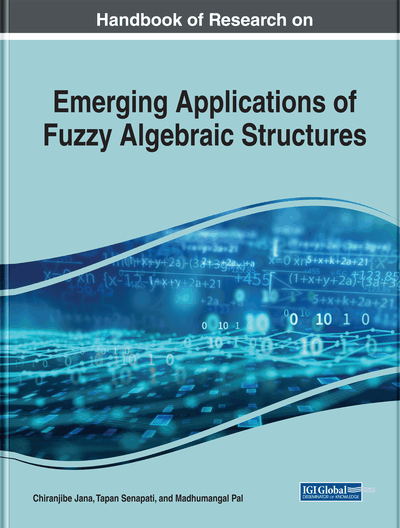An Introduction to Fuzzy Matrices

DOI: 10.4018/978-1-7998-0190-0.ch001
Available
\$37.50
No Current Special Offers

Abstract

Fuzzy matrices are successfully used when fuzzy uncertainty occurs in a problem. Fuzzy matrices have become popular in the last two decades. In this chapter, two new binary fuzzy operators, ⊕ and ⨀, for fuzzy matrices are introduced. Several properties on ⊕ and ⨀ are presented here. Also, some results on existing operators along with these new operators are presented. In this chapter, Hamming and Euclidean distances are introduced for fuzzy matrix and investigated a number of properties. Here, one application is mentioned to recognize a printed character automatically with the help of Hamming distance. The proposed method is applicable when the characters are printed with same font, same size, and same shape. In the case of hand-written characters, the proposed method is also equally effective if it is modified. A lot of works have been done in fuzzy matrices, but there is a huge scope to work with these matrices.
Chapter Preview
Top

2 Preliminaries

The definition of a fuzzy matrix is given below.

Definition 2.1 Fuzzy Matrix. A fuzzy matrix (FM) A of order m×n.is defined aswhere aijμ.is the membership value of the element aij.in A. For simplicity, we write A as A=[aijμ].

A special type of fuzzy matrix called Boolean fuzzy matrix is defined below.

Definition 2.2 Boolean Fuzzy Matrix. A fuzzy matrix.is said to be a Boolean fuzzy matrix of order m×n if all the elements of A are either 0 or 1.

In classical matrix A=[aij] the elements are either any real numbers or complex numbers. The basic arithmetic operators are +,-,*,/ and some mathematical functions. The classical matrices are defined only on real and complex fields. No geometric representation is possible as the elements may be very large and small also.

Fuzzy matrix is the extension of classical matrix. Following issues are new in fuzzy matrix and these issues lead fuzzy matrix a new area of fuzzy mathematics.

• 1.

Many new operators are defined

• 2.

The elements are taken from different fields

• 3.

Can be represented by geometrically

• 4.

Several verities of fuzzy matrices are available, like fuzzy sets

Complete Chapter List

Search this Book:
Reset